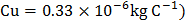# If 100 kWh of energy is consumed at 66 V in a copper voltmeter, then the mass of copper liberated will be (Given ,ECE ofa) 1.65 kg b) 1.8 kg c) 3.3 kg d) 3.6 kg

## Question ID - 151506 :- If 100 kWh of energy is consumed at 66 V in a copper voltmeter, then the mass of copper liberated will be (Given ,ECE ofa) 1.65 kg b) 1.8 kg c) 3.3 kg d) 3.6 kg

3537

 (b) Energy,orkg.

Next Question :

In the circuit shown in the figure, if the potential at pointis taken to be zero, the potential at pointisa)b)c)d)